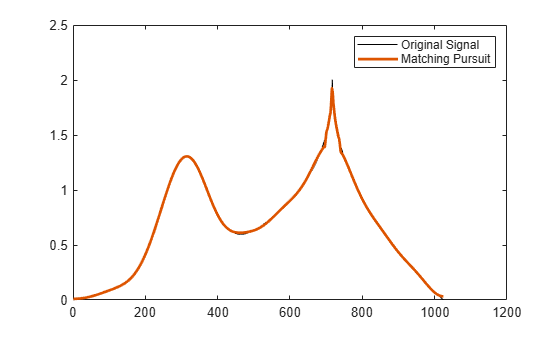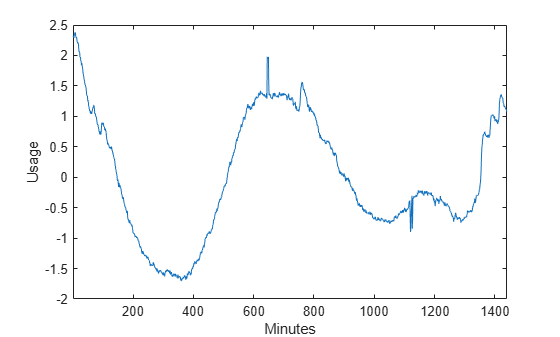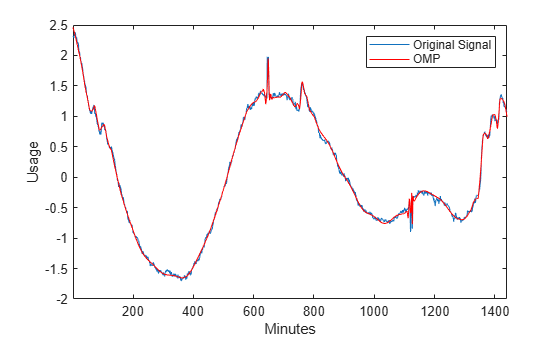Documentation

# wmpalg

Matching pursuit

## Syntax

```YFIT = wmpalg(MPALG,Y,MPDICT) [YFIT,R] = wmpalg(...) [YFIT,R,COEFF] = wmpalg(...) [YFIT,R,COEFF,IOPT] = wmpalg(...) [YFIT,R,COEFF,IOPT,QUAL] = wmpalg(...) [YFIT,R,COEFF,IOPT,QUAL,X] = wmpalg(...) [YFIT,R,COEFF,IOPT,QUAL,X] = wmpalg(...,Name,Value) ```

## Description

`YFIT = wmpalg(MPALG,Y,MPDICT)` returns an adaptive greedy approximation, `YFIT`, of the input signal, `Y`, in the dictionary, `MPDICT`. The adaptive greedy approximation uses the matching pursuit algorithm, `MPALG`. The dictionary, `MPDICT`, is typically an overcomplete set of vectors constructed using `wmpdictionary`.

```[YFIT,R] = wmpalg(...)``` returns the residual, `R`, which is the difference vector between `Y` and `YFIT` at the termination of the matching pursuit.

```[YFIT,R,COEFF] = wmpalg(...)``` returns the expansion coefficients, `COEFF`. The number of expansion coefficients depends on the number of iterations in the matching pursuit.

```[YFIT,R,COEFF,IOPT] = wmpalg(...)``` returns the column indices of the retained atoms, `IOPT`. The length of `IOPT` equals the length of `COEFF` and is determined by the number of iterations in the matching pursuit.

```[YFIT,R,COEFF,IOPT,QUAL] = wmpalg(...)``` returns the proportion of retained signal energy, `QUAL`, for each iteration of the matching pursuit. `QUAL` is the ratio of the ℓ2 squared norm of the expansion coefficient vector, `COEFF`, to the ℓ2 squared norm of the input signal, `Y`.

```[YFIT,R,COEFF,IOPT,QUAL,X] = wmpalg(...)``` returns the normalized dictionary, `X`. `X` contains the unit vectors in the ℓ2 norm corresponding to the columns of `MPDICT`.

```[YFIT,R,COEFF,IOPT,QUAL,X] = wmpalg(...,Name,Value)``` returns an adaptive greedy approximation with additional options specified by one or more `Name,Value` pair arguments.

## Input Arguments

 `MPALG` Matching pursuit algorithm as a character vector or string scalar. Valid entries are: `'BMP'` — Basic matching pursuit`'OMP'` — Orthogonal matching pursuit`'WMP'` — Weak orthogonal matching pursuit Default: `'BMP'` `MPDICT` Matching pursuit dictionary. `MPDICT` is a N-by-P matrix where N is equal to the length of the input signal, `Y`. You can construct `MPDICT` using `wmpdictionary`. In matching pursuit, `MPDICT` is commonly a frame, or overcomplete set of vectors. You may use the Name-Value pair `'lstcpt'` to specify a dictionary instead of using `MPDICT`. If you specify a value for `'lstcpt'`, `wmpalg` calls `wmpdictionary`. `Y` Signal for matching pursuit. `Y` is 1-D, real-valued row or column vector. The row dimension of `MPDICT` must match the length of `Y`.

### Name-Value Pair Arguments

Specify optional comma-separated pairs of `Name,Value` arguments. `Name` is the argument name and `Value` is the corresponding value. `Name` must appear inside quotes. You can specify several name and value pair arguments in any order as `Name1,Value1,...,NameN,ValueN`.

 `'itermax'` Positive integer fixing the maximum number of iterations of the matching pursuit algorithm. If you do not specify a `'maxerr'` value, the number of expansion coefficients, `COEFF`, the number of dictionary vector indices, `IOPT`, and the length of the `QUAL` vector equal the value of `'itermax'`. Default: `25` `'lstcpt'` A cell array of cell arrays with valid subdictionaries. This name-value pair is only valid if you do not input a dictionary in `MPDICT`. Each cell array describes one subdictionary. Valid subdictionaries are: A valid Wavelet Toolbox™ orthogonal or biorthogonal wavelet family short name with the number of vanishing moments and an optional decomposition level and extension mode. For example, `{'sym4',5}` denotes the Daubechies least-asymmetric wavelet with 4 vanishing moments at level 5 and the default extension mode `'per'`. If you do not specify the optional number level and extension mode, the decomposition level defaults to 5 and the extension mode to `'per'`.A valid Wavelet Toolbox orthogonal or biorthogonal wavelet family short name preceded by `wp` with the number of vanishing moments and an optional decomposition level and extension mode. For example, `{'wpsym4',5}` denotes the Daubechies least-asymmetric wavelet packet with 4 vanishing moments at level 5. If you do not specify the optional number level and extension mode, the decomposition level defaults to 5 and the extension mode to `'per'`.`'dct'` Discrete cosine transform-II basis. The DCT-II orthonormal basis is: `${\varphi }_{k}\left(n\right)=\left\{\begin{array}{ll}\frac{1}{\sqrt{N}}\hfill & k=0\hfill \\ \sqrt{\frac{2}{N}}\mathrm{cos}\left(\frac{\pi }{N}\left(n+\frac{1}{2}\right)k\right)\hfill & k=1,2,\dots ,N-1\hfill \end{array}$``'sin'` Sine subdictionary. The sine subdictionary is: `${\varphi }_{k}\left(t\right)=\mathrm{sin}\left(2\pi kt\right)\text{\hspace{0.17em}}\text{\hspace{0.17em}}\text{\hspace{0.17em}}\text{ }k=1,2,\dots ⌈\frac{N}{2}⌉\text{\hspace{0.17em}}\text{\hspace{0.17em}}\text{\hspace{0.17em}}\text{ }0\le t\le 1$``'cos'` Cosine subdictionary. The cosine subdictionary is `${\varphi }_{k}\left(t\right)=\mathrm{cos}\left(2\pi kt\right)\text{\hspace{0.17em}}\text{\hspace{0.17em}}\text{\hspace{0.17em}}\text{ }k=1,2,\dots ⌈\frac{N}{2}⌉\text{\hspace{0.17em}}\text{\hspace{0.17em}}\text{\hspace{0.17em}}\text{ }0\le t\le 1$``'poly'` Polynomial subdictionary. The polynomial subdictionary is: `${p}_{n}\left(t\right)={t}^{n-1}\text{\hspace{0.17em}}\text{\hspace{0.17em}}\text{\hspace{0.17em}}\text{ }n=1,2,\dots 20\text{\hspace{0.17em}}\text{\hspace{0.17em}}\text{\hspace{0.17em}}\text{ }\text{\hspace{0.17em}}\text{\hspace{0.17em}}\text{\hspace{0.17em}}\text{ }0\le t\le 1$``'RnIdent'` The shifted Kronecker delta subdictionary. The shifted Kronecker delta subdictionary is: `${\varphi }_{k}\left(n\right)=\delta \left(n-k\right)\text{\hspace{0.17em}}\text{\hspace{0.17em}}\text{\hspace{0.17em}}\text{ }k=0,1,\dots N$` If you use the `'lstcpt'` name-value pair to generate your dictionary, you can use the additional `'addbeg'` and `'addend'` name-value pairs to append and addend dictionary atoms. See `wmpdictionary` for details. `'maxerr'` Cell array containing the name of the norm and the maximum relative error in the norm expressed as a percentage. Valid norms are `'L1'`, `'L2'`, and `'Linf'`. The relative error expressed as a percentage is `$100\frac{‖R‖}{‖Y‖}$`where R is the residual at each iteration and Y is the input signal. For example, `{'L1',10}` sets maximum acceptable ratio of the L1 norms of the residual to the input signal to 0.10. If you specify `'maxerr'`, the matching pursuit terminates when the first of the following conditions is satisfied: The number of iterations reaches the minimum of the length of the input signal, `Y`, or 500: `min(length(Y),500)`The relative error falls below the percentage you specify with the `'maxerr'` name-value pair. `'stepplot'` Number of iterations between successive plots. `'stepplot'` requires a positive integer. This name-value pair is only valid when `'typeplot'` is 2 or 3 (`'movie'` or `'stepwise'`). `'typeplot'` Type of plot to produce during the progression of matching pursuit. Valid entries for `'typeplot'` are: `0` or `'none'`, `1` or `'one'`, `2` or `'movie'`, `3` or `'stepwise'`. When `'typeplot'` is `'movie'` or `'stepwise'`, the plot updates based on the value of `'stepplot'`. Default: `0` or `'none'` `'wmpcfs'` Optimality factor for weak orthogonal matching pursuit. The optimality factor is a real number in the interval (0,1]. This name-value pair is only valid when `MPALG` is `'WMP'`. Default: `0.6`

## Output Arguments

 `YFIT` Adaptive greedy approximation of the input signal, `Y`, in the dictionary `R` Residual after matching pursuit terminates `COEFF` Expansion coefficients in the dictionary. The selected dictionary atoms weighted by the expansion coefficients yield the approximated signal, `YFIT`. `IOPT` Column indices of the selected dictionary atoms. Using the column indices in `IOPT` with the expansion coefficients in `COEFF`, you can form the approximated signal, `YFIT`. `QUAL` Proportion of retained signal energy for each iteration in the matching pursuit. `QUAL` is a vector with each element equal to `$\frac{||{\alpha }_{k}|{|}_{2}^{2}}{||Y|{|}_{2}^{2}}$`where αk is the vector of expansion coefficients after the k-th iteration. `X` The normalized matching pursuit dictionary. `X` is an N-by-P matrix where N is the length of the input signal, `Y`. The columns of `X` have unit norm.

## Examples

collapse all

Approximate the `cuspamax` signal with the dictionary using orthogonal matching pursuit.

Use a dictionary consisting of `sym4` wavelet packets and the DCT-II basis.

```load cuspamax; mpdict = wmpdictionary(length(cuspamax),'LstCpt',... {{'wpsym4',2},'dct'}); yfit = wmpalg('OMP',cuspamax,mpdict); plot(cuspamax,'k'); hold on; plot(yfit,'linewidth',2); legend('Original Signal',... 'Matching Pursuit');```Obtain the expansion coefficients in the dictionary, the column indices of the selected dictionary atoms, and the proportion of retained signal energy.

Create a dictionary consisting of `sym4` wavelet packets and the DCT-II basis. Approximate the `cuspamax` signal with the dictionary using orthogonal matching pursuit.

```load cuspamax; mpdict = wmpdictionary(length(cuspamax),'LstCpt',... {{'wpsym4',2},'dct'}); [yfit,r,coeff,iopt,qual] = wmpalg('OMP',cuspamax,mpdict);```

This example shows how to set the maximum number of iterations of the orthogonal matching pursuit to 50.

```load cuspamax; lstcpt = {{'wpsym4',1},{'wpsym4',2},'dct'}; mpdict = wmpdictionary(length(cuspamax),'LstCpt',lstcpt); [yfit,r,coeff,iopt,qual] = wmpalg('OMP',cuspamax,mpdict,... 'itermax',50);```

This example shows how to allow for a suboptimal choice in the update of the orthogonal matching pursuit. Relax the requirement to be 0.8 times the optimal assignment. Plot the results stepwise and update the plot every 5 iterations.

```load cuspamax; lstcpt = {{'wpsym4',1},{'wpsym4',2},'dct'}; mpdict = wmpdictionary(length(cuspamax),'LstCpt',lstcpt); [yfit,r,coeff,iopt,qual] = wmpalg('WMP',cuspamax,... mpdict,'wmpcfs',0.8, 'typeplot','stepwise','stepplot',3);```

Obtain a matching pursuit of electricity consumption measured every minute over a 24-hour period.

Load and plot data. The data shows electricity consumption sampled every minute over a 24-hour period. Because the data is centered, the actual usage values are not interpretable.

```load elec35_nor; y = signals(32,:); plot(y); xlabel('Minutes'); ylabel('Usage'); set(gca,'xlim',[1 1440]);```Construct a dictionary for matching pursuit consisting of the Daubechies' extremal-phase wavelet with 2 vanishing moments at level 2, the Daubechies' least-asymmetric wavelet with 4 vanishing moments at levels 1 and 4, the discrete cosine transform-II basis, and the sine basis.

```dictionary = {{'db4',2},'dct','sin',{'sym4',1},{'sym4',4}}; [mpdict,nbvect] = wmpdictionary(length(y),'lstcpt',dictionary);```

Implement orthogonal matching pursuit to obtain a signal approximation in the dictionary. Use 35 iterations. Plot the result.

```[yfit,r,coef,iopt,qual] = wmpalg('OMP',y,mpdict,'itermax',35); plot(y); hold on; plot(yfit,'r'); xlabel('Minutes'); ylabel('Usage'); legend('Original Signal','OMP','Location','NorthEast'); set(gca,'xlim',[1 1440]);```Using the expansion coefficients in `coef` and the atom indices in `iopt`, construct the signal approximation, `yhat`, directly from the dictionary. Compare `yhat` with `yfit` returned by `wmpalg`.

```[~,I] = sort(iopt); X = mpdict(:,iopt(I)); yhat = X*coef(I); max(abs(yfit-yhat))```
```ans = 2.2204e-15 ```

## References

 Cai, T.T. and Wang,L. “Orthogonal Matching Pursuit for Sparse Signal Recovery with Noise”. IEEE® Transactions on Information Theory, vol. 57, 7, 4680–4688, 2011.

 Donoho, D., Elad, M., and Temlyakov, V. “Stable Recovery of Sparse Overcomplete Representations in the Presence of Noise”. IEEE Transactions on Information Theory. Vol. 52, 1, 6–18, 2004.

 Mallat, S. and Zhang, Z. “Matching Pursuits with Time-Frequency Dictionaries”. IEEE Transactions on Signal Processing, vol. 41, 12, 3397–3415, 1993

 Tropp, J.A. “Greed is good: Algorithmic results for sparse approximation”. IEEE Transactions on Information Theory, 50, pp. 2231–2242, 2004.